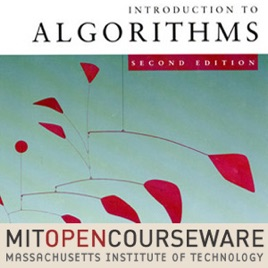23 Folgen

This course teaches techniques for the design and analysis of efficient algorithms, emphasizing methods useful in practice. Topics covered include: sorting; search trees, heaps, and hashing; divide-and-conquer; dynamic programming; amortized analysis; graph algorithms; shortest paths; network flow; computational geometry; number-theoretic algorithms; polynomial and matrix calculations; caching; and parallel computing.# Introduction to Algorithms (2005) MIT

• Technologie

This course teaches techniques for the design and analysis of efficient algorithms, emphasizing methods useful in practice. Topics covered include: sorting; search trees, heaps, and hashing; divide-and-conquer; dynamic programming; amortized analysis; graph algorithms; shortest paths; network flow; computational geometry; number-theoretic algorithms; polynomial and matrix calculations; caching; and parallel computing.

• video
Lecture 01: Administrivia/Introduction/Analysis of Algorithms, Insertion Sort, Mergesort

## Lecture 01: Administrivia/Introduction/Analysis of Algorithms, Insertion Sort, Mergesort

• 1 Std. 20 Min.
• video
Lecture 02: Asymptotic Notation/Recurrences/Substitution, Master Method

## Lecture 02: Asymptotic Notation/Recurrences/Substitution, Master Method

• 1 Std. 10 Min.
• video
Lecture 03: Divide-and-Conquer: Strassen, Fibonacci, Polynomial Multiplication

## Lecture 03: Divide-and-Conquer: Strassen, Fibonacci, Polynomial Multiplication

• 1 Std. 8 Min.
• video
Lecture 04: Quicksort, Randomized Algorithms

## Lecture 04: Quicksort, Randomized Algorithms

• 1 Std. 20 Min.
• video
Lecture 05: Linear-time Sorting: Lower Bounds, Counting Sort, Radix Sort

## Lecture 05: Linear-time Sorting: Lower Bounds, Counting Sort, Radix Sort

• 1 Std. 16 Min.
• video
Lecture 06: Order Statistics, Median

## Lecture 06: Order Statistics, Median

• 1 Std. 8 Min.

4.0 von 5
24 Bewertungen

24 Bewertungen